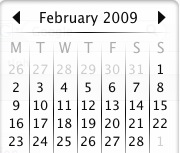#### You may also like### Exploring Wild & Wonderful Number Patterns

EWWNP means Exploring Wild and Wonderful Number Patterns Created by Yourself! Investigate what happens if we create number patterns using some simple rules.### Sending Cards

This challenge asks you to investigate the total number of cards that would be sent if four children send one to all three others. How many would be sent if there were five children? Six?### Dice and Spinner Numbers

If you had any number of ordinary dice, what are the possible ways of making their totals 6? What would the product of the dice be each time?

# It Figures

##### Age 7 to 11Challenge Level

I am writing this on St. Valentine's Day (Feb 14th), and we're half way through the month.

So we're one-and-a-half months into the new year.Well, I know that $1 \frac{1}{2}$ is $\frac{1}{2}$ of $3$, which is $\frac{1}{2}$ of $6$, and that's $\frac{1}{2}$ of $12$.

So what we have is $\frac{1}{2}$ of $\frac{1}{2}$ of $\frac{1}{2}$ of $12$ months.

Now that's $\frac{1}{8}$ of a year!

We can put that down as: $\frac{1}{2}\times \frac{1}{2}\times\frac{1}{2} = \frac{1}{8}$

As the days go by, so the fractions change.

Back in the middle of January we were $\frac{1}{2}$ of $\frac{1}{3}$ of $\frac{1}{4}$ through the year!

$\frac{1}{2}\times \frac{1}{3} \times \frac{1}{4} = \frac{1}{24}$

At the end of February a half of a third through the year:

$\frac{1}{2}$ of $\frac{1}{3}= \frac{1}{6}$

Well, let's explore the interesting numbers we get.

Suppose we allow ourselves to use three numbers less than $10$ and multiply them together [like I used $2$, $2$, $2$, for the middle of February and $2$, $3$, $4$, for the middle of January].

Then we could get things like :-

$2 \times 3 \times 5 = 30$
$3 \times 5 \times 8 = 120$
$6 \times 6 \times 6 = 216$

and so on ...

See if you can find lots and lots.

You could then explore these numbers in different ways.

A usual thing is to put them in order from the lowest to the highest.

You could look at the answers that come up more than once. Like $36$ which comes up three times from doing :-

$2 \times 3 \times 6$
$2 \times 2 \times 9$
$3 \times 3 \times 4$

When you find things out from these answers that you get you should ask yourself WHY??

Now we always should ask ourselves "I wonder what would happen if I ...?".

So, "I wonder what would happen if we only allowed ourselves to use two numbers below $10$ to multiply together?"

Now we will get answers like :-

$7 \times 2 = 14$
$3 \times 8 = 24$
$5 \times 9 = 45$

Now if you find $31$ different answers I thought you will have found them all!
However thanks to Mr A Thompson from Chestnutgrove School Academy in Wandsworth and his hard working Yr 7 students , I stand corrected and the number is $46$!!

Like before, we could write them out in order and explore them. Don't forget to look at those answers that occur more than once like $18$ which comes from :-

$2 \times 9$
$3 \times 6$

Well some of you will now be aware that there are $120$ answers altogether from multiplying three figures together. [I wonder how you know that? Was it just by counting? Did you do some interesting additions? Did you ...?]

We're counting the ones that maybe have the same answer but have come from different multiplications.

When you only have two figures to multiply together then there are $36$ answers.

How have you done?

Can you find the rest?

So what on earth happens if we use four or even more figures to multiply together?

Or maybe you'd like to use numbers higher than $9$ [perhaps up to $12$] allowing you, when using three numbers altogether to have:

$2 \times 5 \times 12 = 120$
$11 \times 11 \times11 = 1331$